# Difference between regular functions and arrow functions

This article discusses the major differences between the regular functions and the arrow functions.

Arrow functions – a new feature introduced in ES6 – enable writing concise functions in JavaScript. While both regular and arrow functions work in a similar manner, yet there are certain interesting differences between them, as discussed below.

Syntax

Syntax of regular functions:-

 `let x = ``function` `function_name(parameters){ ` `   ``// body of the function ` `}; `

Example of regular functions:-

 `let square = ``function``(x){ ` `  ``return` `(x*x); ` `}; ` `console.log(square(9)); `

Output: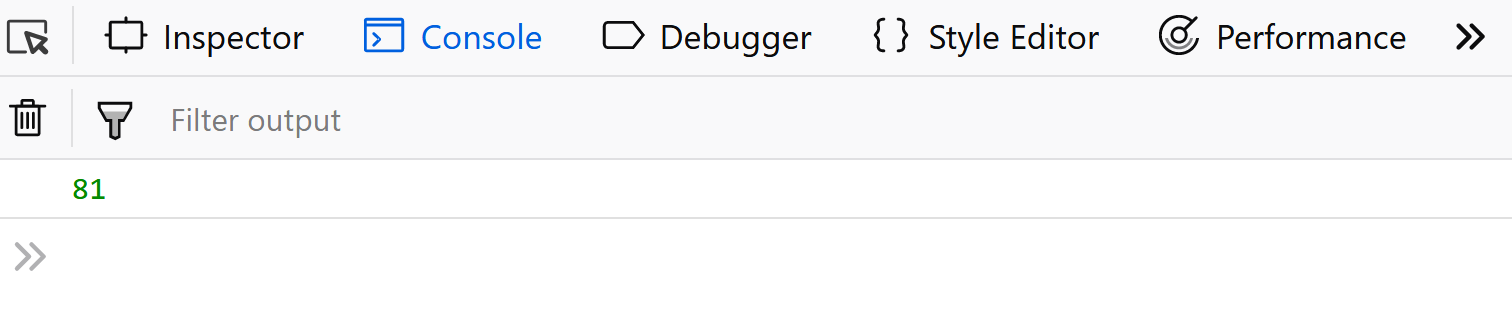The syntax of arrow functions:-

 `let x = (parameters) => { ` `    ``// body of the function ` `}; `

Example of arrow functions:-

 `var` `square = (x) => { ` `    ``return` `(x*x); ` `}; ` `console.log(square(9)); `

Output:Use of this keyword

Unlike regular functions, arrow functions do not have their own `this`.
For example:-

 `let user = { ` `    ``name: ``"GFG"``, ` `    ``gfg1:() => { ` `        ``console.log(``"hello "` `+ ``this``.name); ``// no 'this' binding here ` `    ``}, ` `    ``gfg2(){        ` `        ``console.log(``"Welcome to "` `+ ``this``.name); ``// 'this' binding works here ` `    ``}   ` ` ``}; ` `user.gfg1(); ` `user.gfg2(); `

Output: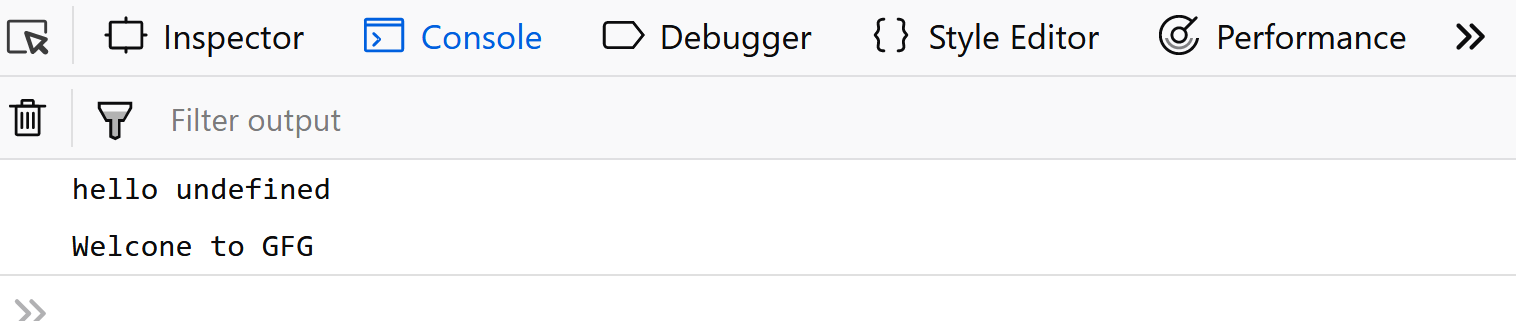Availability of `arguments` objects

Arguments objects are not available in arrow functions, but are available in regular functions.

Example using regular ():-

 `let user = {       ` `    ``show(){ ` `        ``console.log(arguments); ` `    ``} ` `}; ` `user.show(1, 2, 3); `

Output: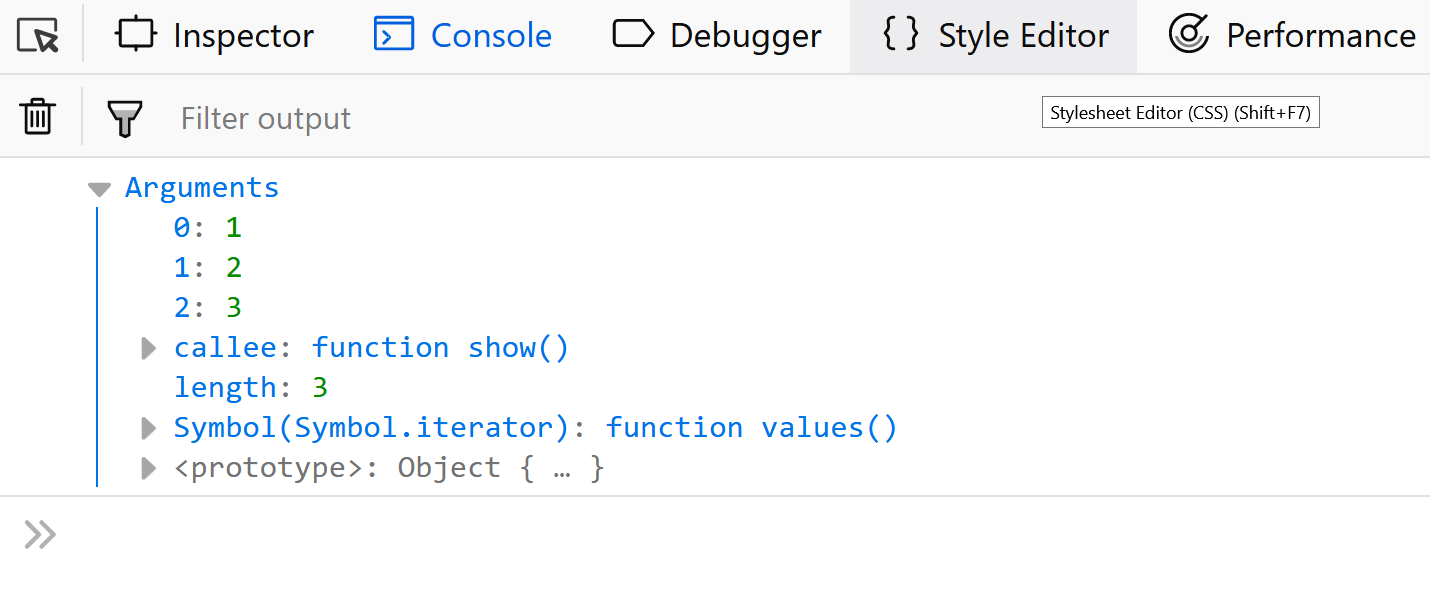Example using arrow ():-

 `let user = {      ` `        ``show_ar : () => { ` `        ``console.log(...arguments); ` `    ``} ` `}; ` `user.show_ar(1, 2, 3); `

Output: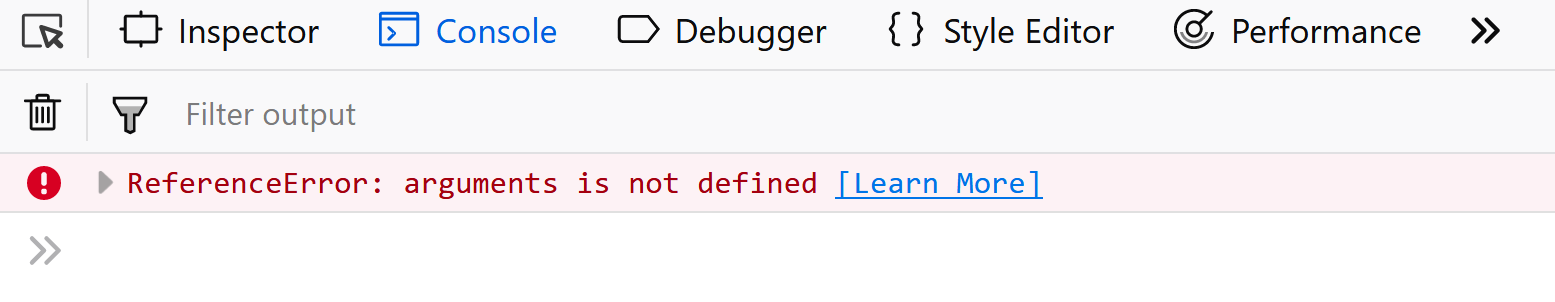Using `new` keyword

Regular functions created using function declarations or expressions are ‘constructible’ and ‘callable’. Since regular functions are constructible, they can be called using the ‘new’ keyword. However, the arrow functions are only ‘callable’ and not constructible. Thus, we will get a run-time error on trying to construct a non-constructible arrow functions using the new keyword.

Example using regular function:-

 `let x = ``function``(){ ` `    ``console.log(arguments); ` `}; ` `new` `x =(1,2,3); `

Output: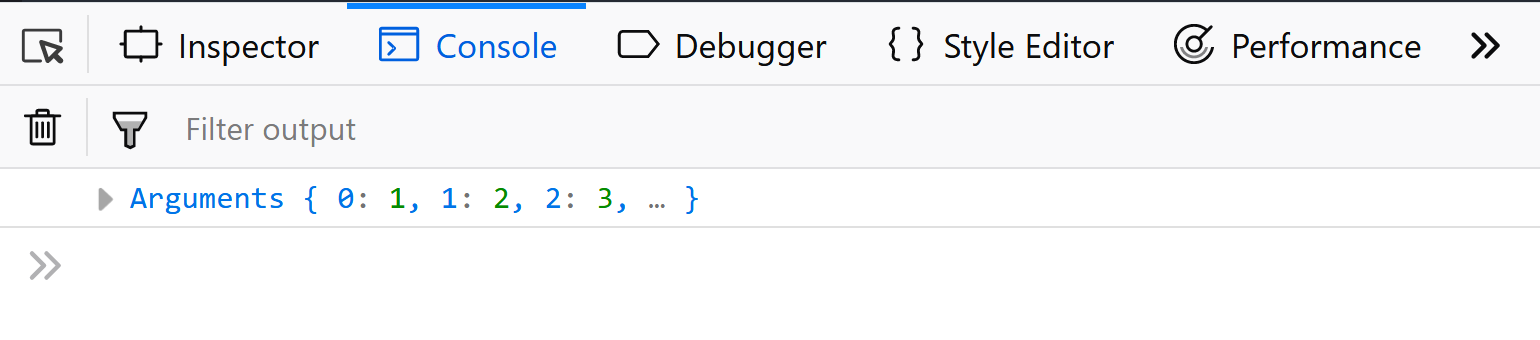Example using arrow function:-

 `let x = ()=> { ` `    ``console.log(arguments); ` `}; ` `new` `x(1,2,3); `

Output: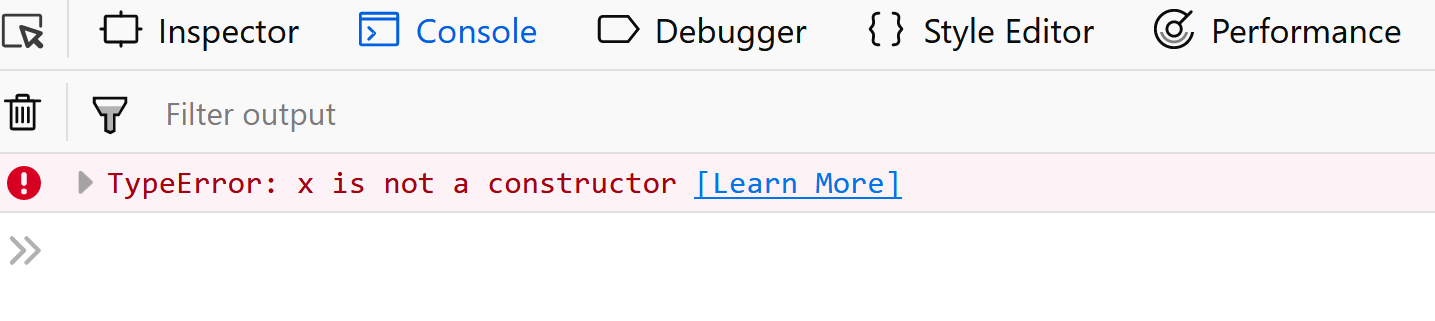My Personal Notes arrow_drop_upCheck out this Author's contributed articles.

If you like GeeksforGeeks and would like to contribute, you can also write an article using contribute.geeksforgeeks.org or mail your article to contribute@geeksforgeeks.org. See your article appearing on the GeeksforGeeks main page and help other Geeks.

Please Improve this article if you find anything incorrect by clicking on the "Improve Article" button below.

Improved By : shubham_singh

Article Tags :

6

Please write to us at contribute@geeksforgeeks.org to report any issue with the above content.